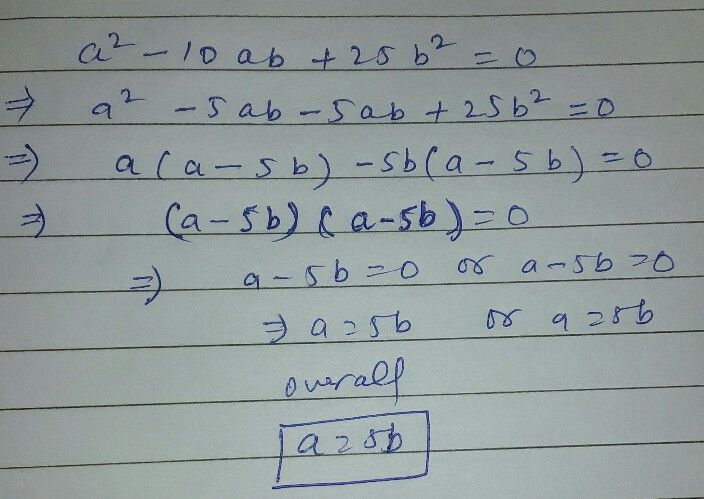Symbol
Problem$93.$ $a^{2}$ $→$ $8ab$ $+16b^{2}=0$ 0 66. $a^{2}$ $→$ $10ab$ $+25b^{2}=0$ 57. $y=\sqrt{6x-5}$ oyHKUHÉ